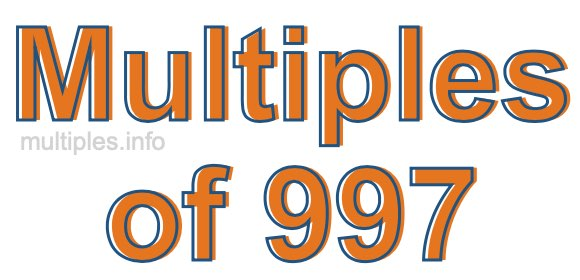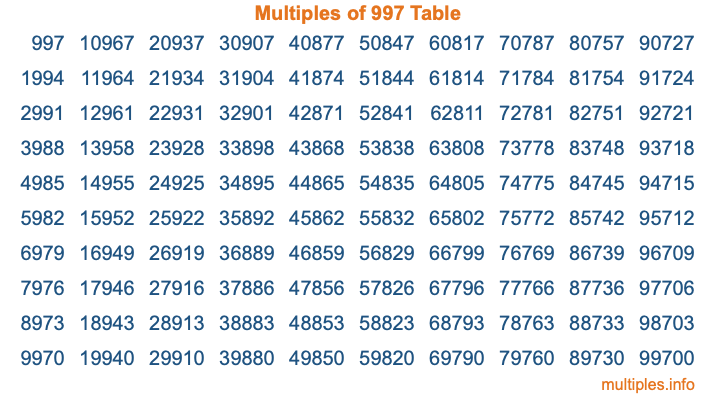Multiples of 997Welcome to the Multiples of 997 page. Here we will first teach you everything you will ever need to know about the multiples of 997, and then give you a study guide summary of everything we taught you to make sure you remember it all. Use this page to look up facts and learn information about the multiples of 997. This page will make you a multiples of nine hundred ninety-seven expert!

Definition of Multiples of 997
Multiples of 997 are all the numbers that when divided by 997 equal an integer. Each of the multiples of 997 are called a multiple. A multiple of 997 is created by multiplying 997 by an integer.

Therefore, to create a list of multiples of 997, you start with 1 multiplied by 997, then 2 multiplied by 997, then 3 multiplied by 997, and so on for as long as you want. Thus, the list of the first five multiples of 997 is 997, 1994, 2991, 3988, and 4985. To see a larger list of multiples of 997, see the printable image of Multiples of 997 further down on this page. We also have a category where you can choose any nth multiple of 997.

Multiples of 997 Checker
The Multiples of 997 Checker below checks to see if any number of your choice is a multiple of 997. In other words, it checks to see if there is any number (integer) that when multiplied by 997 will equal your number. To do that, we divide your number by 997. If the the quotient is an integer, then your number is a multiple of 997.

Is  a multiple of 997?

Least Common Multiple of 997 and ...
A Least Common Multiple (LCM) is the lowest multiple that two or more numbers have in common. This is also called the smallest common multiple or lowest common multiple and is useful to know when you are adding our subtracting fractions. Enter one or more numbers below (997 is already entered) to find the LCM.

Check out our LCM Calculator if you need more details about the Least Common Multiple or if you need the LCM for different numbers for adding and subtraction fractions.

nth Multiple of 997
As we stated above, 997 is the first multiple of 997, 1994 is the second multiple of 997, 2991 is the third multiple of 997, and so on. Enter a number below to find the nth multiple of 997.

th multiple of 997

Multiples of 997 vs Factors of 997
997 is a multiple of 997 and a factor of 997, but that is where the similarities end. All postive multiples of 997 are 997 or greater than 997. All positive factors of 997 are 997 or less than 997.

Below is the beginning list of multiples of 997 and the factors of 997 so you can compare:

Multiples of 997: 997, 1994, 2991, 3988, 4985, etc.

Factors of 997: 1, 997

As you can see, the multiples of 997 are all the numbers that you can divide by 997 to get a whole number. The factors of 997, on the other hand, are all the whole numbers that you can multiply by another whole number to get 997.

It's also interesting to note that if a number (x) is a factor of 997, then 997 will also be a multiple of that number (x).

Multiples of 997 vs Divisors of 997
The divisors of 997 are all the integers that 997 can be divided by evenly. Below is a list of the divisors of 997.

Divisors of 997: 1, 997

The interesting thing to note here is that if you take any multiple of 997 and divide it by a divisor of 997, you will see that the quotient is an integer.

Multiples of 997 Table
Below is an image of the first 100 multiples of 997 in a table. The table is in chronological order, column by column. The first column has the first ten multiples of 997, the second column has the next ten multiples of 997, and so on.The Multiples of 997 Table is also referred to as the 997 Times Table or Times Table of 997. You are welcome to print out our table for your studies.

Negative Multiples of 997
Although not often discussed or needed in math, it is worth mentioning that you can make a list of negative multiples of 997 by multiplying 997 by -1, then by -2, then by -3, and so on, to get the following list of negative multiples of 997:

-997, -1994, -2991, -3988, -4985, etc.

Multiples of 997 Summary
Below is a summary of important Multiples of 997 facts that we have discussed on this page. To retain the knowledge on this page, we recommend that you read through the summary and explain to yourself or a study partner why they hold true.

There are an infinite number of multiples of 997.

A multiple of 997 divided by 997 will equal a whole number.

997 divided by a factor of 997 equals a divisor of 997.

The nth multiple of 997 is n times 997.

The largest factor of 997 is equal to the first positive multiple of 997.

997 is a multiple of every factor of 997.

997 is a multiple of 997.

A multiple of 997 divided by a divisor of 997 equals an integer.

997 divided by a divisor of 997 equals a factor of 997.

Any integer times 997 will equal a multiple of 997.

Multiples of a Number
Here you can get the multiples of another number, all with the same attention to detail as we did for multiples of 997 on this page.

Multiples of
Multiples of 998
Did you find our page about multiples of nine hundred ninety-seven educational? Do you want more knowledge? Check out the multiples of the next number on our list!